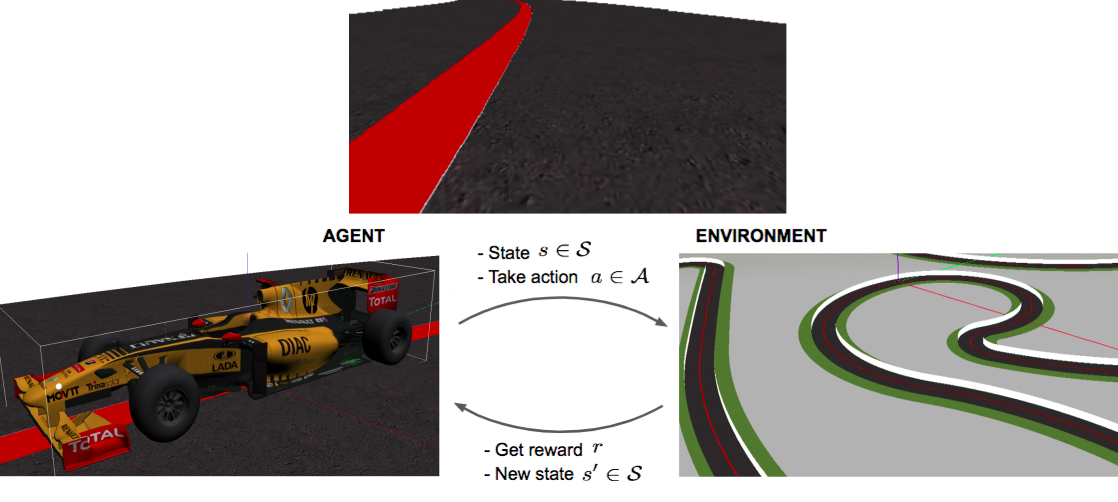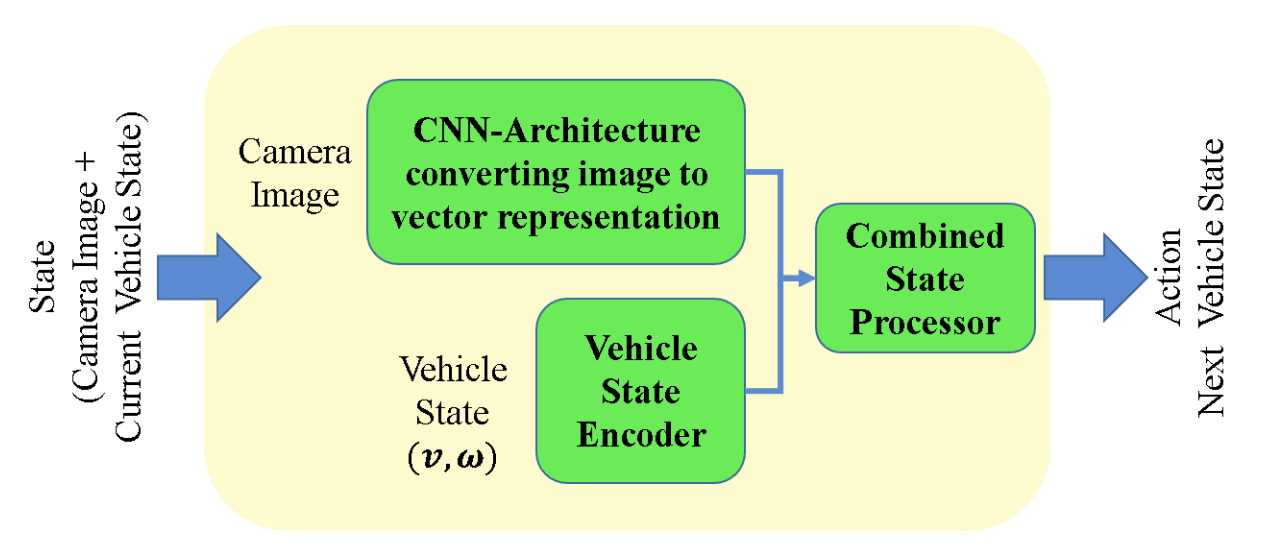# Community Bonding: Week 2

This week was all about analyzing last week’s results, strengthening RL baseline and setting up my remote access at URJC Tamino platform.

## Objectives

• Finalizing the RL Baseline
• Setting Up server for future computations
• Ablation study of Pilot-Net on multiple brains

Reinforcement Learning (RL) is a strategy by learning based on interactions and feedback. Let’s consider an agent in an unknown environment and it can obtain some rewards (positive feedback) by interacting with the environment. Following a fundamental strategy, the agent ought to take actions so as to maximize cumulative rewards. In our case, this scenario is a F1-racing car trying to maximize the cummulative reward by the following the line and moving forward in the race track. A figure showing such an interaction is given below.

The agent a.k.a. the racing car observes an observation i.e. the current state of the front camera image view and decides the linear velocity and angular velocity which is fed into the simulator (Gym Gazebo ) to simulate the dynamics and get the next step.The OpenAI Gym  framework provides libraries to design structured agent-environment settings, interactions and feedbacks. It helps in the design of effective reinforcement learning agents. In the concerned work, a sample custom environment was made with the help of custom environment settings provided by OpenAI Gym. A tyical custom environment architecture consists of:

• Reset function: It resets all the environment variables and prepares the agent and environment for a new episode
• Step function: Given an action $a$ choosen by the RL agent based on a policy $\pi_\theta$ and the current state $s$, i.e. $a \sim \pi_\theta (s)$, this function gives the next observation (state, $s'$), the reward feedback ($r$) and a termination flag ($f=[True, False]$) to inform if the episode is over or not.

## Custom RL pipeline preparation

The pipeline we are concerned of deals with both sensor based observations and the internal state of the robot. So, the overall state-vector for such a setting should consist of information from all of cameras images, laser distances and velocities of the robot. Thus the modified actor for our formulation has the capability of having all kinds of informations together and is shown in the figure below.With the following configuration, the custom environment is designed to give both the images ($o_t$) and the velocity state (${\bf v}_t = [v_t, \omega_t]$). Now the actor policy, $\pi_\theta$, takes ${o_t, {\bf v}_t}$ and passes both of them through image encoder $\phi_o$ and velcoity encoder $\phi_v$, to get output $s_t = \{\phi_o(o_t), \phi_v(v_t, \omega_t)\}$. After getting action, $a_t$, the transition dynamics ($P$) of the environment as simulated in Gym Gazebo provides us with: $o_{t+1}, {\bf v}_{t+1}, r_{t+1}, f_{t+1} \sim P(.|s_t, a_t)$ and
$s_{t+1} = \{\phi_o(o_{t+1}), \phi_v(v_{t+1}, \omega_{t+1})\}$

The current implementation consists of 4 algorithms  in PyTorch:

• Deep Q-Learning: Off-Policy algorithm for discrete action spaces where there are finite choices for the actions to perform i.e. an eample can be (straight, backward, left, right)
• Deep Deterministic Policy Gradient: Off-Policy learning algorithm for continuous action spaces where an actions consists of infinitely many possibilities within a range i.e. for example predicting traget velcoties between 0 m/s to 13 m/s.
• Proximal Policy Optimization: On-Policy learning algorithm which can work for both discrete and continuous actions.

## Setting up Tamino Server

I am grateful to my mentors and the URJC team to provide me access to their Tamino server for all my computational requirements. I feel very positive to have a high computational capacity system with 8 core CPUs and a Nvidia 1080 GPU. So this week was spent in setting up ROS-Noetic and Behavior Metrics into the server system and setting up appropriate visualizations using the guidelines in .

## Simulation Analysis and Results

Just for some visuals, the video below shows the PilotNet brain completing Montreal Circuit and failing at a difficult corner at the Montmelo circuit. Well, this validates the baseline PilotNet on pytorch:

 https://github.com/JdeRobot/BehaviorMetrics/tree/noetic-devel/gym-gazebo
 https://gym.openai.com/
 https://github.com/TheRoboticsClub/gsoc2021-Utkarsh_Mishra/tree/community_bonding/RL_algorithms
 The Arcade Learning Environment: An Evaluation Platform for General Agents, Bellemare et al. 2013
 Continuous Control With Deep Reinforcement Learning, Lillicrap et al. 2016
 Proximal Policy Optimization Algorithms, Schulman et al. 2017
 https://jderobot.github.io/BehaviorMetrics/install/ros_noetic